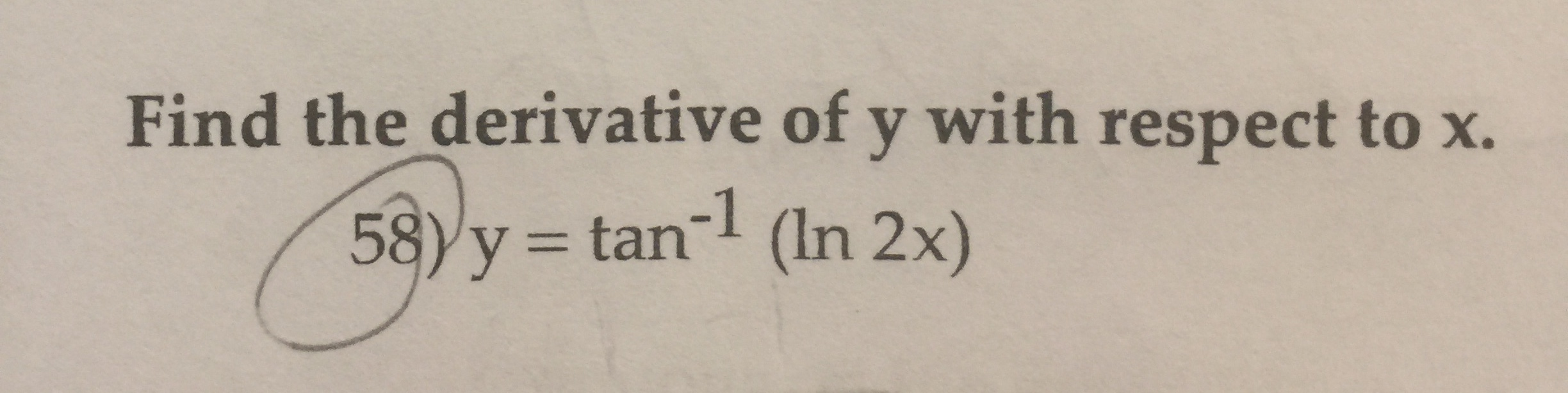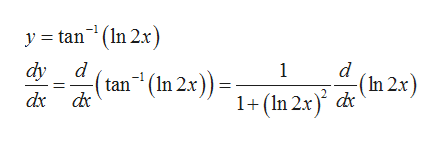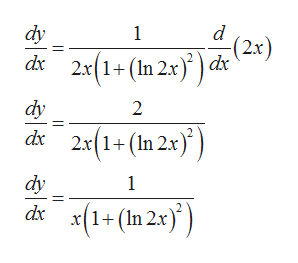# Find the derivative of y with respect to x.58)y tan (In 2x)

Questionhelp_outlineImage TranscriptioncloseFind the derivative of y with respect to x. 58)y tan (In 2x) fullscreen
check_circle

Step 1

Firstly, we use the result that  derivative of inverse of tan of x is 1/1+x^2help_outlineImage Transcriptionclosey tan(n 2x dy d 1 (tan (In 2x)) 1+ (In 2.x) d (In 2x) dx d fullscreen
Step 2

Then we use the result that derivative of lnx is 1/x

Step 3

Then we use the result that d...help_outlineImage Transcriptionclosedy 1 (2x) d 2x(1+(In 2x)d* dy 2 2x(1+ (In 2x) d dy 1 dx(1+(In 2.x) fullscreen

### Want to see the full answer?

See Solution

#### Want to see this answer and more?

Solutions are written by subject experts who are available 24/7. Questions are typically answered within 1 hour.*

See Solution
*Response times may vary by subject and question.
Tagged in

### Calculus